### Seeing is believing!

Before you order, simply sign up for a free user account and in seconds you'll be experiencing the best in CFA exam preparation.

##### Subject 3. Valuation of Default-Free Callable and Putable Bonds
Binomial models are usually constructed to value bonds with embedded options. The process of valuing a bond with embedded options is only slightly more complicated than the one that we have already described. The only notable difference is that we now have to make so-called "nodal decisions" at each node.

Zero Volatility

In the absence of default and interest rate volatility, the bond's future cash flows are certain.

Let's assume that a Bermudan-style bond can be called by the issuer since the end of year 1 at par (price of 100). We use the same backward induction approach to value such bond, but at each node we make a nodal decision, specified by the following formula:

Value at the Node = Min {Value from backward induction, Call price}

This formula is an example of a decision rule. In our case, the rule is very simple - the bond is called whenever the intrinsic value of the call becomes positive (value exceeds call price). So, at each node we compare the value that we get from backward induction and the call price, which is assumed to be par in this example. Whenever the value is higher than the par, we substitute it with par. When the value at the node does not exceed par, we leave it as it is and continue the backward induction process.

Valuation of a callable bond is demonstrated by the following example: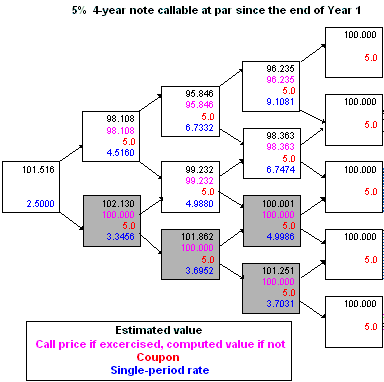According to the stated call rule, the bond is called at the four special nodes, because in these cases the estimated value at the node exceeds par. Let's demonstrate the last step in this backward induction process - calculation of the value at the root of the tree. The one-year rate at the root is 2.5%. If the higher rate (4.516) is effective at the end of year 1, the value at the next node is 98.108. The bond is not expected to be called at this node, because its value is below par. On the contrary, if rates increase by a lesser amount, to 3.3456, the estimated value of the bond at the next node would be 101.229, so the bond would be called, since it is valued above par. We substitute the value at this node with par (call price). We now discount the two values and coupons to find the value at the root:

V0 = 1/2 x (V1, H+ C) / (1 + r0) + 1/2 x (V1, L + C) / (1 + r0) = 1/2 x (98.108 + 5) / (1+0.025) + 1/2 x (100 + 5)/(1+0.025) = 101.516.

In contrast, if the bond is putable, the decision to exercise the option is made by the bondholder, who will exercise the put option when the discounted value of the bond's future cash flows is lower than the put price.

Effect of Interest Rate Volatility

Volatility does not affect the value of a straight bond. However, higher volatility does increase the value of any embedded option, and:

• decreases the value of a callable bond.
• increases the value of a putable bond.

Changes in the level and shape of the yield curve also affect the value of a callable or putable bond.

When interest rates are high, the bond is unlikely to be called. Therefore, the callable bond will have similar price/yield relationship (positive convexity) as a comparable straight bond. As interest rates decline, the value of a straight bond will rise. The value of a callable bond will also rise, but not as much as the straight bond. This is because the value of the call option will rise too:

Value of callable bond ↑ = Value of straight bond ↑ - value of issuer call option ↑

The call price will set an upper limit on the price of the callable bond.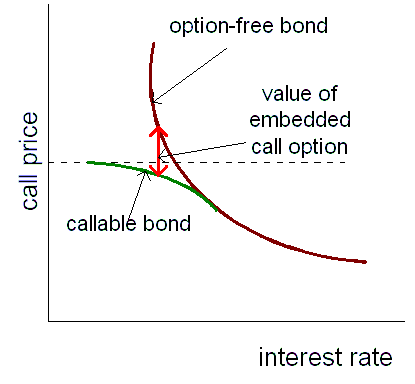As interest rates rise, the value of a straight bond will decline. The value of an otherwise identical putable bond will also decline, but not as much as the straight bond. This is because the value of the put option will rise:

Value of putable bond ↓ = Value of straight bond ↓ + value of investor put option ↑As the yield curve flattens:

• The value of the call option increases.
• The value of the put option decreases.

Valuation

Valuing a bond with embedded options assuming an interest rate volatility requires three steps:

• Generate a tree of interest rates based on the given yield curve and volatility assumptions;
• at each node of the tree, determine whether the embedded options will be exercised; and
• apply the backward induction valuation methodology to calculate the present value of the bond.

Let's see what happens to our interest rate tree based on the same yield curve, when we increase the assumed volatility from 15% to 20%: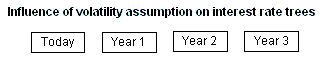15% Annual Volatility Assumption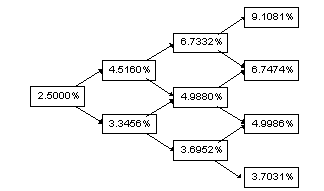20% Annual Volatility Assumption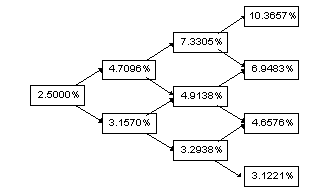As demonstrated by the exhibit above, when yield volatility is higher, the interest rate tree becomes more dispersed. Consequently, there is a greater probability that the values at future nodes will be higher than the call price or lower than the put price. The higher probability of options being exercised makes these options more valuable.

User Comment
americade Interesting point that the greater range makes the embedded options more valuable whether you are short or long the options in the bond. the better point is that volatility increases the value of an option per BS, it is the main input of the six inputs in the black scholes.
mchu octavianus... you must be kidding...
pochuevalex octavarius they are either calculated values from backward induction (if valuepar)
charomano Present value = (Value + Coupon) / (1+yield)

If PV < Par => use PV
If PV > Par => use Par
Ex: Year 3 (Upper part)
96.235 = 0.5 *(100 + 5)/(1+9.1081%) + 0.5*(100+5)/(1+9.1081%)

Since 96.235 < 100 => Use 96.235
Bootstraper Shouldn't it 102.3 instead of 101.229 in the example?
czar Octa, a bond is usually called at par... It can be a bit above or below par, but the underlying reason is, if the bond value is greater than par, then the bond issuer, would rather call back the bond at par...
xiaoniu The embedded call option increases in value with higher interest rate volatility. Therefore, the value of a callable bond decreases with higher volatility.

The embedded put option increases in value with higher interest rate volatility. Therefore, the value of a putable bond increases with higher volatility.

As interest rates decrease, the value of a straight bond increases, and the value of the embedded call option in a callable bond also increases. Because the investor is effectively short on the embedded call option, the value of the callable bond increases less than that of the straight bond, limiting the upside for the investor.
TheCFAGuy no one has preed what octavianus was really asking.

except for the column of prices in the period just before redemption, the bond prices include the option cost and are therefore lower than the price of a straight bond. notice there are a few options where YTM<coupon but the price of the bond is still below par.

the option cost has not been provided and therefore the right response to octa is that you can't calculate those call values - they are for demonstration purposes only. poor octa was probably tearing his hair out calculating the straight bond value.

the reason the prices in the final period are equal to the value of a straight bond is because there is no longer the chance for the issuer to call the bond after that
davidt876 can we all agree that the call price only acts as an upper limit where the issuer explicitly states it won't paid accrued interest when it calls back the bond?

if not the discounted value of the coupon pushes the bond value above the call price. i agree the call price has an effect in terms of the FV value used in the PV calculation, but it's certainly not acting as an upper limit.

for example, if the first callable date is in 1yr; coupon = 5%; call price (FV) = 100; & required rate = 2% then PV = 102.94. regardless of the fact that the bond is callable in 1yr at 100, that future \$5 coupon is so valuable that investors take the hit on the capital side